Shipra Saxena — April 6, 2021

## Objective

• The List is an integral part of python when we talk about data structure.
• Understand how to perform different operations over a list.

## Introduction

Let’s imagine a scenario, we are required to store marks of all the students in class 10th roll number wise. One way to solve this problem is to use variables for all the students and store their marks. As shown below.

Note: If you are more interested in learning concepts in an Audio-Visual format, We have this entire article explained in the video below. If not, you may continue reading.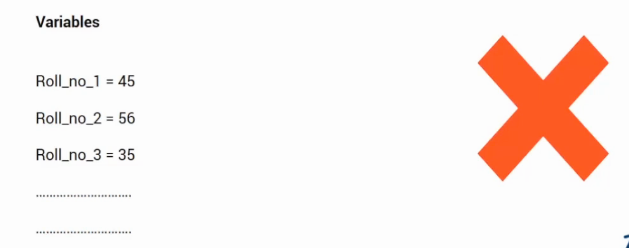But this is not an efficient way to solve our problem as

• The number of variables becomes very large as the number of students increase. This can lead to large memory overhead.
• We will not be able to analyze the data for answering some questions like what are the average marks of the last five students? We can not answer these questions since variables do not inherently support sequence.

So we need a data structure that supports sequences and helps in questions related to data. This is where the list comes in.

In this article, we will be talking about the list. Let’s see the topics we will be covering.

1. What is a List?
2. Sub-setting
3. Adding elements to an existing list
4. Deleting elements
5. Looping over a list to access its elements.

Let’s now discuss each of them in detail.

## What is a List?

A list is an ordered data structure with elements separated by a comma and enclosed within square brackets. For example, list1 and list2 shown below contains a single type of data.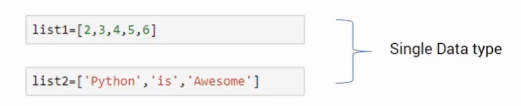Here, list1 has integers while list2 has strings. Lists can also store mixed data types as shown in the list3 here.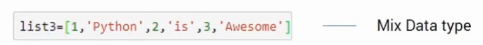## Subsetting

Now let’s see how can we access the elements of a list. Before we subset it, let’s see how the elements are stored in it. Take the example of the list, its elements are stored using indexes starting from 0.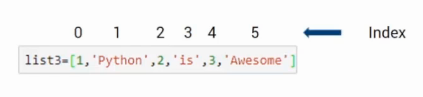Here, element 1 stands at 0, and element 2 stands at index 1, and so on.

If you wish to extract a single element, say at index 1 we can use the square bracket along with the index number. So, list3 will give the “Python”, which is present at index 1. Like this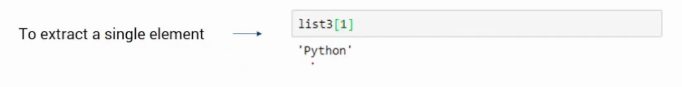This was how we can extract a single element from a list, what if, we want to extract a sequence out of it. We can do this using the following format, where within a square bracket we can give a range separated by a colon.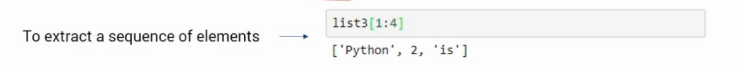If I give, list3[1:4], it will start from index 1 and reaches up to one index before 4. That means the list starts from 1 and stops at 3 it doesn’t include 4. Similarly, if you give list3[2:5] it will return a list of elements in the list3 from index 2 to 4.

Negative indices are another interesting concept. Suppose, you want to access the last element from it, you can use list3[-1]. It will give you the last element in the list which is Awesome in this case.

## Adding Elements to an Existing List

Now let’s explore how can we add an element to an existing list. A single element can be added using an append function. As shown below.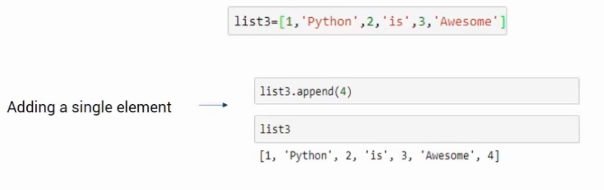In this example using append, we have added element 4 at the end.

We can also add multiple elements to the list, for this we use extend function. The extend function accepts the multiple elements, in comparison to append which accepts a number i.e a single element. Look at the example below-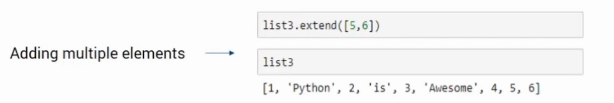Here, the function will extract each element from the input list and add it to the main list which is list3 in this case.

We can also add a list to the existing one. For this, we can use the append function as discussed previously. The only difference is instead of a number we will pass lists as input. It will add the whole input list as an element.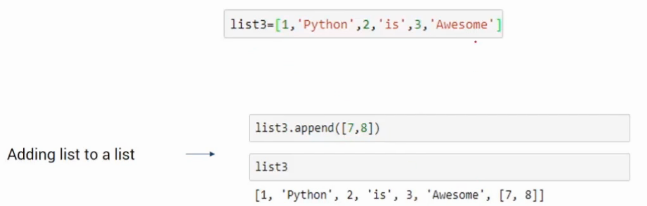So, in this example, the input list [7,8] is added at index 6th in the list3. Also, it can be accessed using the index value.

## Deleting elements

Let’s see how can we remove elements from a given list. Suppose you want to remove an element by its value. In this case, we can use the remove function and giving the element that we want to remove as an input. For example, if we want to remove 2 as shown below, we can use the code list3.remove(2). when you print the list there will be no 2 present in it.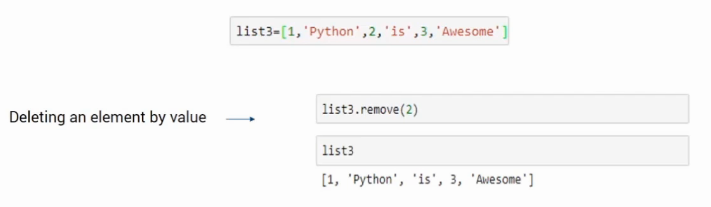The second method is when we use the index value to remove the element from it. In such a case, we will use the del keyword. Suppose I want to remove the element present at index 3, I will use del list3. As shown in the following image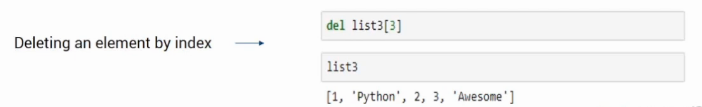## Looping over The List

Let’s see how one can iterate over each element of a list for performing a task such as printing. Here is an example of how we can iterate over it using a For loop to print the elements.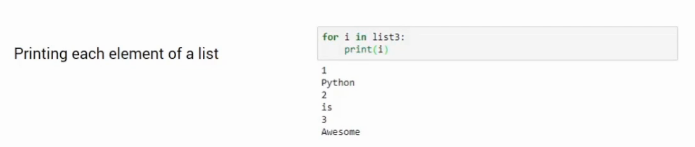The For loop will extract each element and print it. This is how we can get access to each element using a ‘for’ loop.

## End Notes

This was all about Lists in this article. Here, we saw what it is, how to access elements from it. Also, we saw how to add and delete elements from it along with that how to iterate over them to process it further.

If you are looking to kick start your Data Science Journey and want every topic under one roof, your search stops here. Check out Analytics Vidhya’s Certified AI & ML BlackBelt Plus Program

If you have any queries let me know in the comment section!